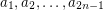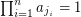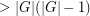# Olson's Conjecture

 Importance: Medium ✭✭
 Author(s): Olson, John E.
 Subject: Number Theory » Combinatorial Number Theory
 Keywords: zero sum
Conjecture   Ifis a sequence of elements from a multiplicative group of order, then there existso that.
A famous theorem of Erdos, Ginzburg, and Ziv asserts that every sequence ofelements from an additive abelian group has a subsequence of lengthwhich sums to. This pretty result has lead to numerous generalizations. In particular, Olsen generalized this result by showing that every sequence ofelements from an arbitrary multiplicative group of orderhas a subsequence of lengthwhich has product equal toin some order. The above conjecture asserts that this reordering is not needed. Apart from Olson's result, there appears to be very little known about this problem. Next we highlight an obvious question which appears untouched.
For every finite multiplicative group, letdenote the smallest integerso that every sequence ofelements ofhas a subsequence of lengthwith product equal toin the given order (so Olsen's conjecture is equivalent to). It is clear that, since any sequence of lengthmust contain at leastcopies of the same element, and the product of these will be. However, I (M. DeVos) don't know how to improve significantly on this upper bound, and it would appear to me that any significant progress in this direction would require a little something new.